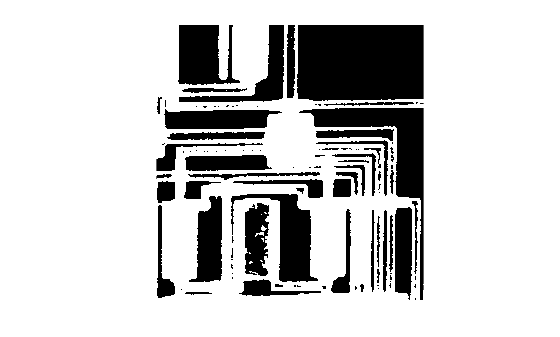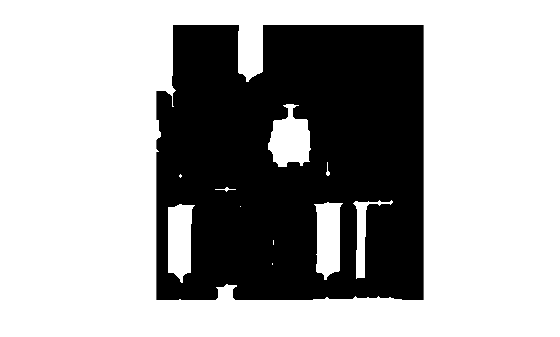Documentation

## Erode an Image To Remove Thin Lines

This example shows how to erode a binary image using the `imerode` function.

Read a binary image into the workspace. Display the image.

```BW1 = imread('circbw.tif'); figure imshow(BW1)```Create a structuring element. The following code creates a diagonal structuring element object.

`SE = strel('arbitrary',eye(7))`
```SE = strel is a arbitrary shaped structuring element with properties: Neighborhood: [7x7 logical] Dimensionality: 2 ```

Erode the image, specifying the input image and the structuring element as arguments to the `imerode` function.

`BW2 = imerode(BW1,SE);`

Display the original image and the eroded image. Notice the diagonal streaks on the right side of the output image. These are due to the shape of the structuring element.

```figure imshow(BW2)```